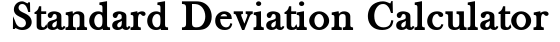##### Related Content

Programmers and Teachers:Nikkolas
Tutor and degreed Aerospace Engineer

# Standard Deviation CalculatorData is from:

## Standard Deviation Lesson

### What is the Standard Deviation?

In statistics, the standard deviation is a measure of how dispersed the values in a data set are. It is the square root of the variance. The formula for population standard deviation is given as:

$$\sigma ={\sqrt {{\frac {1}{N}}\sum _{i=1}^{N}(x_{i}-\mu )^{2}}}$$

And the formula for sample standard deviation is given as:

$$s ={\sqrt {{\frac {1}{N-1}}\sum _{i=1}^{N}(x_{i}-\bar{x} )^{2}}}$$

Where N is the number of values in the data set, xi is the data point of index i, μ is the population mean, and is the sample mean.

### Why we Calculate the Standard Deviation of a Data Set

A large standard deviation means the values are very dispersed, on average the difference between values is large. A small standard deviation means the values are not very dispersed and have small differences between them on average.

We can create a distribution chart based on the dispersion of a data set. For example, we use the standard deviation to validate if a data set is normally distributed. A set is normally distributed if most of the data falls within 1, 2, and 3 standard deviations of the mean (see empirical rule).

## How the Calculator Works

The standard deviation calculator on this page is written in the programming language JavaScript (JS). Since JS runs in your device’s internet browser, there is no waiting on communications to a server or page refreshes. Therefore, you get your answer nearly instantly.

When you click the “calculate” button, the inputted data set gets formatted into a JS array. That array is then fed to a library of math functions, where the actual standard deviation calculation is performed. After rounding the answer, the standard deviation is printed to the output area in the calculator.

Scroll to Top